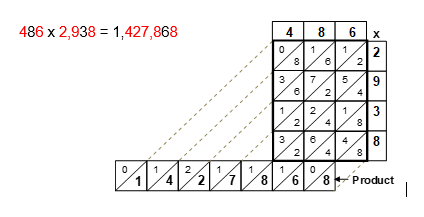Select Page

Each month, a new set of puzzles will be posted.  Come back next month for the solutions and a new set of puzzles, or subscribe to have them sent directly to you.

## MIND-Xpander maths problems

1. The second of five consecutive multiples of 11 is removed. The other four are then added together to give 715. What is the lowest multiple of the remaining four? There are two possible solutions.
2. Simplify the following expression: √5 x √15 x √10

## PRIME Numbers puzzle

Throwing 3 darts at the following dartboard and all 3 throws must hit a number on the dartboard, what are your chances that their total will equal a Prime Number? Numbers can be used more than once.## HEXAGON-numeric puzzle (Level 1)

Fit the numbers 1 – 6 in each hexagon and where the hexagon segments touch each other, the numbers in these segments will be the same. No number can be repeated in a hexagon. Find the remaining missing numbers to complete the following puzzle.## Feedback

There are more than one way of doing these puzzles and may well be more than one answer.  Please let me and others know what alternatives you find by commenting below.  We also welcome general comments on the subject and any feedback you'd like to give.

If you have a question that needs a response from me or you would like to contact me privately, please use the contact form.

## Get more puzzles!

If you've enjoyed doing the puzzles, consider ordering the books;

• Book One - 150+ of the best puzzles
• Book Two - 200+ with new originals and more of your favourites

Both in a handy pocket sized format.   Click here for full details.

## MIND-Xpander puzzle

You have a piece of paper, 10 cm. by 10 cm. Area = 100 cm². For some reason, you need a square piece of paper with an area of 50 cm². Using the piece of paper, you have, what’s an easy way of getting the new square?

Solution## Algebraic Challenge puzzle

By replacing each question mark with an algebraic sign (+, -, x and /), solve the following equations for the answers as shown:

(6 ? 4) ? 12 = 12

(7 ? 3) ? (4 ? 1) = 26

(4 ? 2) ? (4 ? 3) = 24

(6 ? 5) ? (9 ? 2) = 19

Solution

(6 x 4) – 12 = 12

(7 x 3) + (4 + 1) = 26

(4 / 2) x (4 x 3) = 24

(6 – 5) + (9 x 2) = 19

## Chinese Multiplication puzzle

Also known as a lattice method, the Chinese method provides an easy way to multiply any two numbers without the aid of a calculator. In the following puzzles, some or part of the multiplied or added values are provided. The object of this puzzle is to find the missing values to determine the original numbers that when multiplied together gives you the correct product as shown on the bottom line. Find these missing values.

Solution